# RS Aggarwal Class 6 Solutions Chapter 12 Parallel Lines Ex 12

## RS Aggarwal Class 6 Solutions Chapter 12 Parallel Lines Ex 12

These Solutions are part of RS Aggarwal Solutions Class 6. Here we have given RS Aggarwal Solutions Class 6 Chapter 12 Parallel Lines Ex 12.

Question 1.
Solution:
In the given figure, the pairs of parallel edges are :
AB || CD and BC || AD

Question 2.
Solution:
In the given figure, pairs of all possible parallel edges are:
AB | | HE | | DC || GF ; BE | | CF || AH
| | DG ; AD | | GH | | BC | | EF

Question 3.
Solution:
(i) In the given figure,
DE || BC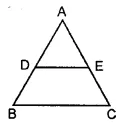(ii) In the given figure,
AB || DC ; AD || BC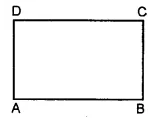(iii) In the given figure,
AB || DC ; AD || BC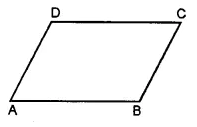(iv) In the given figure,
LM || RQ, MP || RS, PQ || SL.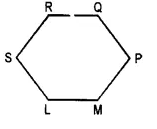(v) In the given figure,
AB || CD, CD || EF, AC || BD, CE || DF, AB || EF.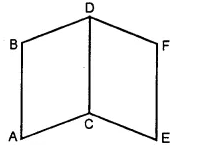Question 4.
Solution:
(i) Place the rular so that one of its measuring edges lies along the line. Hold it firmly with one hand. Now place a set square with one arm of the right angle coinciding with the edge of the rular. Read off the distance between i and m on the set square which is 1.7cm.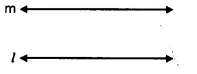(ii) Place the rular so that one of the measuring edges of the rular lies along the line /. Hold it firmly with one hand and place a set square with one arm of the right angle coinciding with the edge of the rular. Read off the distance between the lines / and m on the set square which is 1.2 cm.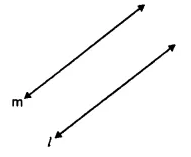Question 5.
Solution:
It is given that l || m.
Also AB ⊥ l i.e. AB is the perpendicular distance between two parallel lines l and m.
Again CD ⊥ l i.e. CD is the perpendicular distance between two parallel lines l and m.
But the perpendicular distance between two parallel lines is always same everywhere.
CD = AB = 2.3 cm.

Question 6.
Solution:
In the given figure, we see that the line segments AB and CD do not intersect. But, the corresponding lines determined by them will clearly intersect. So, the segment AB and CD are not parallel.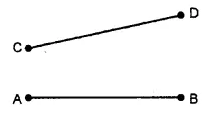Question 7.
Solution:
(i) Place the rular so that one of its measuring edges lies along of its measuring edges lies along the line l. Hold it firmly and place a set square with one arm of the right angle coinciding with the edge of the rular. Draw the line segment AB along the edge of the set square as shown in figure.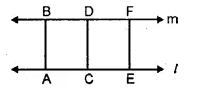Slide the set square along the rular and draw some more segments CD and EF. We observe that AB = CD = EF.
l || m.
(ii) Place the rular so that one of its measuring edges lies along the line l. Hold it firmly and place a set square with one arm of the right angle conciding with the edge of the rular. Draw the line segment AB along the edge of the set square.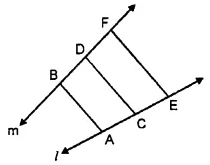Slide the set square along the rular and draw some more segments CD and EF as shown in the figure.
We observe that AB ≠ CD ≠ EF
Hence l is not parallel to m.

Question 8.
Solution:
(i) True
(ii) True
(iii) False
(iv) False.

Hope given RS Aggarwal Solutions Class 6 Chapter 12 Parallel Lines Ex 12 are helpful to complete your math homework.

If you have any doubts, please comment below. Learn Insta try to provide online math tutoring for you.